# GSEB Solutions Class 9 Maths Chapter 7 Triangles Ex 7.3

Gujarat Board GSEB Solutions Class 9 Maths Chapter 7 Triangles Ex 7.3 Textbook Questions and Answers.

## Gujarat Board Textbook Solutions Class 9 Maths Chapter 7 Triangles Ex 7.3

Question 1.
ΔABC and ΔDBC are two isosceles triangles on the same base BC and vertices A and D are on the same side of BC (see figure). If AD is extended to intersect BC at P, show that
1. ΔABD ≅ ΔACD
2. ΔABP ≅ ΔACP
3. AP bisects ∠A as well as ∠D
4. AP is the perpendicular bisector of BC.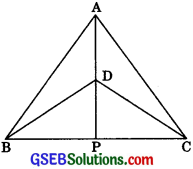Solution:
1. In ΔABD and ΔACD,
AB = AC (given) (ΔABC is an isosceles triangle)
BD = CD (given) (ΔDBC is an isosceles triangle)
∴ ΔABD ≅ ΔACD (by SSS congruency)

2. In ΔABP and ΔACP,
AB = AC (given)
∠BAP = ∠CAP (CPCT as AABD ≅ ∠ACD)
AP = AP (common)
∴ ΔABP ≅ ΔACP (by SAS congruency)

3. ΔABP ≅ ΔACP (Proved in part (ii))
BP = CP ………(1) (byCPCT)
and ∠BAP = ∠CAP (by CPCT)
∴ AP bisect ∠A.
In ΔBDP and ΔCDP,
BD = CD (given ΔBDC is an isosceles triangle)
BP = CP (Proved above in part (ii))DP = DF (Common)
∠BDP = ∠CDP (by SSS congruency)
Hence ∠BDP = ∠CDP (by CPCT)
∴ AP bisect ∠D.

4. Since ΔBDP≅ΔCDP (Proved above)
BP = CP (by CPCT)
∠BPD = ∠CPD (by CPCT)
∠BPD + ∠CPD = 180° (linear pair)
∴ ∠BPD + ∠BPD = 180°
2∠BPD = 180°
∠BPD = 90°
∴ AP is the perpendicular bisector of BC.

Question 2.
AD is an altitude of an isosceles triangle ABC in which AB = AC. Show that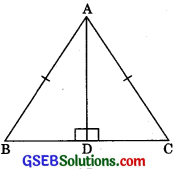solution:
AB = AC (ΔABC is an isosceles triangle)
∴ ΔABD ≅ ΔACD (by RHS congruency)
BD = CD (by CPCT)Question 3.
Two sides AB and BC and median AM of one triangle ABC are respectively equal to sides PQ and QR and median PN of åPQR (see figure). Show that
(i) ΔABM ≅ ΔPQN
(ii) ΔABC ≅ ΔPQR.
Solution:
Given In ΔABC and ΔPQR,
AB = PQ
BC = QR
and AM = PN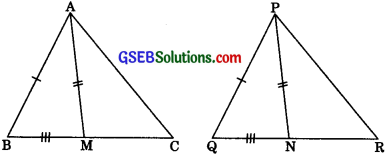(i) In ΔABM and ΔPQN,
AB = PQ (given)
BC = QR (given)
∴ $$\frac {1}{2}$$BC = $$\frac {1}{2}$$QR (Dividing by 2)
Hence BM = QN (median bisect opposite sides)
AM = PN
∴ ΔABM ≅ ΔPQN (by SSS congruency)
∠ABM = ∠PQN (by CPCT)
⇒ ∠ABC = ∠PQR

(ii) In ΔABC and ΔPQR,
AB = PQ (given)
∠ABC = ∠PQR (proved above in part (i))
BC = QR (Given)
∴ ΔABC ≅ ΔPQR (by SAS congruency)Question 4.
BE and CF are two equal altitudes of a triangle ABC. Using RHS congruence rule, prove that the triangle ABC is isosceles.
Solution:
Given: ΔABC in which BE = CF.
To Prove: ΔABC is an isosceles triangle
Proof: In ABEC and ΔCFB,
∠BEC = ∠CFB (each 900)
BC = CB (Common)
BE = CF (given)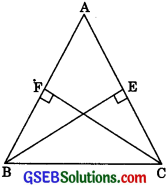∴ ∠BEC = ∠CFB (by RHS congruency)
∴ ∠CBF = ∠BCE (by CPCT)
Hence AB = AC (Sides opposite to equal angle are equal)
Therefore, ABC is an isosceles triangle.

Question 5.
ABC is an isoscele8 triangle with AB = AC. Draw AP ⊥ BC to show that ∠B = ∠C.
Solution:
Given: ABC is an isosceles triangle in which
AB = AC.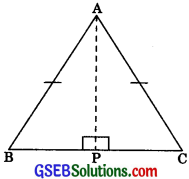To Prove: ∠B = ∠C
Construction: Draw AP ⊥ BC
Proof: In right triangles APB and APC,
∠APB = ∠APC (each 90°)
AB = AC (given)
AP = AP (common)
∴ ΔAPB ≅ ΔAPC (By RHS congruency)
∠ABP = ∠ACP (by CPCT)
⇒ ∠B = ∠C